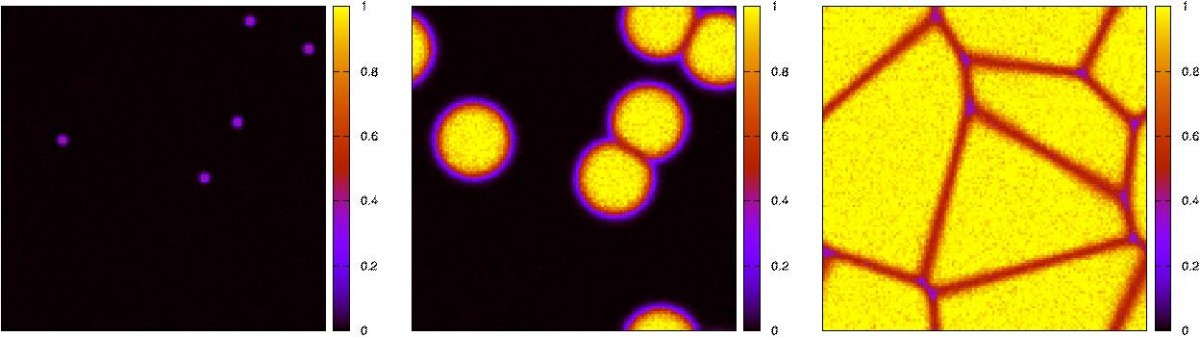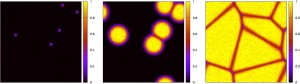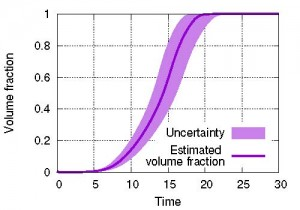Data assimilation for massive autonomous systems based on a second-order adjoint methodShin-ichi Ito, Hiromichi Nagao, Akinori Yamanaka, Yuhki Tsukada, Toshiyuki Koyama, Masayuki Kano, and Junya Inoue

Physical Review E 94, 043307 (2016), https://doi.org/10.1103/PhysRevE.94.043307

Abstract

Data assimilation (DA) is a fundamental computational technique that integrates numerical simulation models and observation data on the basis of Bayesian statistics. Originally developed for meteorology, especially weather forecasting, DA is now an accepted technique in various scientific fields. One key issue that remains controversial is the implementation of DA in massive simulation models under the constraints of limited computation time and resources. In this paper, we propose an adjoint-based DA method for massive autonomous models that produces optimum estimates and their uncertainties within reasonable computation time and resource constraints. The uncertainties are given as several diagonal elements of an inverse Hessian matrix,which is the covariancematrix of a normal distribution that approximates the target posterior probability density function in the neighborhood of the optimum. Conventional algorithms for deriving the inverse Hessian matrix require O(CN2 + N3) computations and O(N2) memory, where N is the number of degrees of freedom of a given autonomous system and C is the number of computations needed to simulate time series of suitable length. The proposed method using a second-order adjoint method allows us to directly evaluate the diagonal elements of the inverse Hessian matrix without computing all of its elements. This drastically reduces the number of computations to O(C) and the amount of memory to O(N) for each diagonal element. The proposed method is validated through numerical tests using a massive two-dimensional Kobayashi phase-field model. We confirm that the proposed method correctly reproduces the parameter and initial state assumed in advance, and successfully evaluates the uncertainty of the parameter. Such information regarding uncertainty is valuable, as it can be used to optimize the design of experiments.